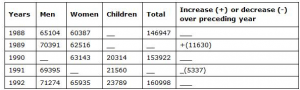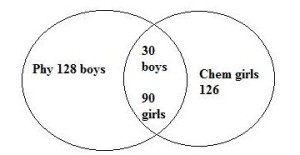## Data Interpretation For SBI PO : Set -43

D.1-5) Following table gives the population of a locality from 1988 to 1992. Read the table and answer the questions.1) The number of children in 1998 is

a) 31236

b) 125491

c) 14546

d) 21456

e) None of these

d)

The number of children in 1988 = Total Population – (Men +women)

146947 – (65104+60387)

146947 – 125491 = 21456

2) The total population in 1989 is

a) 144537

b) 158577

c) 146947

d) 149637

e) None of these

b) Total Population in 1989

Population in 1988 + increase

146947+ 11630 = 158577

3) Number of children in 1989 is

a) 25670

b) 14040

c) 13970

d) 15702

e) None of these

a)

Number of children in 1989

Total population in 1989 – Total Population of men and women

158577 – (70391+62516) = 25670

4) Number of women in 1991 is

a) 57630

b) 56740

c) 52297

d) 62957

e) None of these

a)

Number of women in 1991

Total Population in 1991 – total population in men and women in 1991

(153922- 5337) – (69395 + 21560) = 57630

5) Increase or decrease of population in 1992 over 1991 is

a) – (12413)

b) + (12413)

c) + 155661

d) + 7086

e) None of these

b)

Total Population in 1992 = 160998

Total Population in 1991 = 153922 – 5337 = 148585

Increase in population in 1992 over 1991

160998-148585 = 12413

D.6-10) Study the information carefully to answer the given questions

In a college out of 600 students, boys and girls are in the ratio 8: 7. Each students went to either  physics lab or chemical lab or both, 40% of the boys attend only physics lab, total 20% children went  to both the labs out of which 25% are boys, 45% of the girls went to only chemistry lab.

6) Total how many boys went to only physics lab ?

a) 175

b) 158

c)156

d)134

e) None of these

b)8:7 = 320(boys):280(girls)

20% both = 20*600/100 = 120

B – (phy ) = 40*320/100 = 128

B – (both) = 25*120/100 = 30

G – (chem) = 45*280/100 = 126

Total no of boys(phy) = 128+30 = 158

7) How many girls went to only physics lab ?

a) 62

b) 46

c) 64

d) 60

e) None of these

c)

No of girls (phy) = (280-126 – 90) = 64

8) Total number of students went to physics lab is what percentage of the total number of students in the college

a) 22%
b) 32%
c) 33%
d) 36%

e) None of these

b)

Total no of students went to only phy = 128+64 = 192

% = 192*100/600 = 32%

9)  What is the total number of girls went to chemistry lab ?

a) 216

b) 210

c) 267

d) 261

e) None of these

a)

Total no of Girls(chem) = 126+90 = 216

10) What is the total number of students went to only chemistry lab ?

a) 244

b) 248

c) 284

d) 288

e) None of these